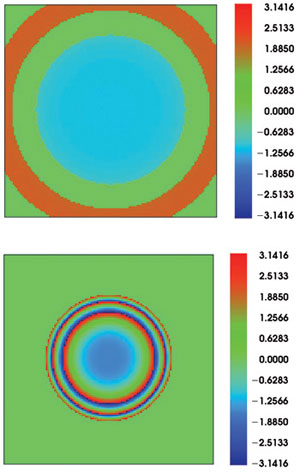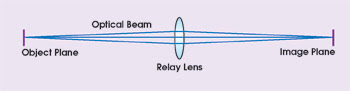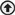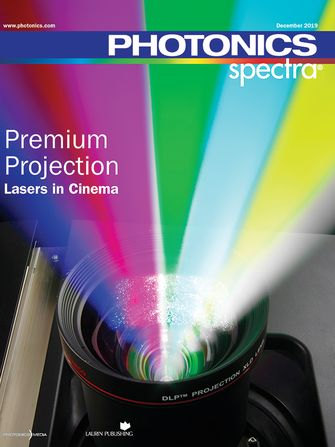# Physical and Geometric Methods Combined to Model Optics

A hybrid lens design uses diffraction to model beam propagation and geometrical optics theory to compute the effects of arbitrary lenses.

Kenneth E. Moore

For nearly the entire history of modern lens design, engineers have used geometric ray tracing to model light propagation through optical components. This may soon change, however, thanks to the ever-increasing speed of computers.

Ray tracing is based on the assumption that light is essentially a multitude of independent incoherent particles. Decomposing the beam into incoherent rays means that diffraction or coherent interference between parts of the beam is not an issue. Thus, the lens designer need consider only two cases: propagation of the particles between optical surfaces and the transfer of the particles through those surfaces. Whatever the case, the particles travel along paths called rays.

Proven though the technique may be, the ray model is basically an approximation made primarily to avoid the computational overhead and algorithm complexity of more rigorous propagation models. Faster computers have essentially eliminated the overhead problem, thrusting algorithm development into the spotlight for many researchers. There even has been recent discussion about how best to extend the ray-based design tools, or whether to replace them with a new set of computational or hybrid tools.1,2

One alternative is to use a physical model for optics propagation, but this has some problems. As with geometric ray tracing, designers must consider two basic components. The beam will propagate between optical surfaces and will transfer from one side of a surface to the other. The difference is that the most convenient representation of the beam is a two-dimensional array of points, with the complex amplitude of the electric field stored at each point in the array.

Storing arrays

This system allows storing two arrays, one for X-direction and one for Y-direction polarization. If polarization is unimportant, a single array will suffice.

The array can have a different number of points in the two orthogonal directions. Point-to-point spacing also can vary. To efficiently compute the required fast Fourier transform of the array, it is desirable to use a power of two for the number of points along each direction. Typical array sizes are on the order of 128, 256 or 512 points. The primary limitations on the number of points in the array are computer memory and computation time.

For example, with an unpolarized double-precision complex-amplitude array, every data point requires 16 bytes. A 256 x 256 array would therefore use 1 Mb of RAM; a 1024 x 1024 array would use 16 Mb. Polarized beams require twice as much RAM as unpolarized beams.

When building the propagation model, defining the input beam requires some knowledge of its source. Typical sources would be Gaussian laser beams, uniform-amplitude top-hat beams or beams computed from prior physical optics propagations. The definition of the beam also depends upon where the analysis will begin in the optical system. One common starting point is the entrance pupil. The methods described here work for any scalar beam, independent of the amplitude or phase distribution of the electric field.

Physical optics propagation theory begins with a number of simplifying assumptions. For the majority of optical lens systems, the Fourier propagation theory is a good compromise between accuracy and practicality.3,4 Fresnel theory assumes that the beam travels primarily along a local axis and that one can ignore the vector nature of the electric field (that is, the theory is scalar diffraction).

It also assumes a small beam extent compared with propagation length. When this assumption cannot be made, an alternate theory called angular spectrum propagation will work. This is fortunate because it is common for lenses to have propagation lengths much shorter than the beam extent; for example, in light propagation between closely spaced lens elements. One plus for both Fresnel and angular spectrum propagation is that required theoretical development and computational algorithms are well-known.5

Exploring hybrid design

When working with a model based on physical optics propagation, the difficult stage in the lens design process is that of light propagation through the optical surfaces. Their curvature is often quite steep relative to the curvature of the isophase wavefront of the beam. Rigorous modeling of the diffraction propagation of an electric field through an arbitrary surface shape would require the application of complex boundary conditions on the electric field. Although such computations may be practical with planar or even spherical surfaces where symmetry may be exploited, almost all practical applications in modern lens design involve skew (nonaxial) beams with arbitrary phase and amplitude incident upon nonspherical surfaces.Figure 1.
Above is a phase display for a lens system with a pinhole aperture where the center of the beam shows a uniform, nearly perfect flat-phase profile. The red band is energy-deposited in the first Airy ring. Hybrid modeling is necessary because a traditional geometrical analysis alone would be unable to predict the effects of spatial filtering by the pinhole. Below are the beam aberrations before they were designed out of the system.

One solution is for the lens designer to play off the strength of both models (Figure 1). In Focus Software’s Zemax design software, for example, the design process reverts to ray tracing to transfer the beam through an optical surface. Recall that the propagation of rays along straight lines ignores the coherent diffraction interference between various parts of the beam. However, this effect is most pronounced with long propagation lengths. For very short ones, the geometric theory is more reliable. The self-interference effect is also negligible if the rays are far apart; for instance, far from focus. Geometric theory also has the advantage over physical optics modeling in that it applies to any optical surface, including planes, spheres and arbitrary shapes, even when no symmetry exists. Ray tracing is fast, and there is no need to develop new algorithms and surface models.

To account for light propagation through optical surfaces, the first step in the hybrid lens design technique involves computing a transfer function using ray tracing and then applying the function to the physical beam.

Computation is as follows:

• The software assumes that the beam travels by diffraction propagation until the center of the beam contacts the optical surface. For the moment, the calculations ignore any possible intersection of the beam with the surface, so the propagation in this sense may be partially virtual.

• The system determines a spherical input reference surface that best fits the phase of the beam at that point.

• The next step is to calculate the radius of curvature of the output reference sphere by tracing small differential rays from near the center of the beam, normal to the input reference sphere, and through the optical surface.

• After the system establishes a grid of rays on the input surface, with all ray vectors pointing along the local normal of the input reference surface, it traces the rays through the surface, where they propagate to the output reference surface.

Designers can now compute the geometric transfer function by evaluating the ray data on the output reference sphere. The software can glean the following effects from the ray trace and apply them collectively as an operator to the physical optics beam:

• The phase along each ray yields optical aberrations.

• The complex amplitude transmission of the ray (if a polarization ray trace is performed) produces attenuation and polarization phase effects.

• The variation in spacing of the rays yields local anamorphic compression or expansion of the beam, resulting in increases or decreases in complex amplitude.

• Vignetting of the rays essentially zeros out the altitude transmission, in an effect similar to the common Kirchhoff boundary condition.

Note that it is possible to extend the concept of the geometrical transfer function to multiple surfaces at once. There is no need to ray trace through just one surface. Applications for this further simplification of the model include propagating through relatively thin lenses or windows, or through media where the Fresnel theory is not adequate but where the ray model suffices.

Once it applies the complex geometric transfer function to the beam, the software performs another Fresnel or angular spectrum propagation to bring the beam to the next optical surface. Calculations continue until the algorithm reaches the last surface.

Spatial filtering

To explore the hybrid modeling process, consider a 1:1 relay system with a 200-mm object-to-lens distance, 200-mm lens-to-object distance and 50-mm lens diameter. Optimization of the lens with a conic asphere would provide perfect geometric imaging with these parameters.

Place a Gaussian beam with a 5-μm waist radius and a 0.6328-μm wavelength at the object plane, set the beam’s initial peak irradiance to 1 W/mm2, and the beam will diverge up to about 8.06 mm at the lens, well within the lens aperture. A physical optics analysis will then reveal the beam at the image surface. The beam will focus to a nearly perfect Gaussian shape at the object plane (Figure 2). The small decrease in peak irradiance is due to the finite aperture of the lens. The geometric transfer function detects the vignetting of the outermost rays and sets the amplitude transmittance of that portion of the transfer function to zero. A smaller lens aperture will show a more pronounced effect.Figure 2.
With a Gaussian beam having a 5-μm waist radius and a 0.6328-μm wavelength placed at the object plane, and initial peak irradiance of that beam set to 1 W/mm2, the beam for this 1:1 relay system will focus to a nearly perfect Gaussian profile at the object plane.

To add aberrations to this system, designers can include 1.0 wave of spherical aberration at the 8-mm radial aperture to the front surface of the lens. By zeroing in on the phase as it propagates just past the aberrated lens, the software displays concentric rings of aberration in the beam (Figure 1). With the phase referenced to a sphere centered on the focal point 200 mm away from the beam, the center of the phase shows a value of approximately 21.57 rad, the Gaussian beam’s Gouy shift.5 This aberrated beam then propagates 200 mm to the original focus plane. Because of the spherical aberration, the best focus is slightly closer to the lens, but the use of paraxial focus simplifies the discussion a bit.

This hybrid lens design method can compute the beam at any plane, not just near focus (Figure 3). When examining the new cross section of the beam at focus, it can show an obvious deviation from the ideal Gaussian distribution, with a decrease in Strehl ratio (relative peak irradiance) and substantial spreading of the beam.Figure 3.
The hybrid lens design method can compute the beam parameters at any plane, not just near focus. Examination of one result at focus indicates a decrease in relative peak irradiance and substantial deviation from Gaussian.

With a 10-μm-diameter aperture comparable to the unaberrated Gaussian waist size placed at the focus, the software displays a truncated beam. Back-propagate the beam to 200 mm, and the center shows a uniform, nearly perfect flat-phase profile. The green circular band indicates a region where intensity is below the 1 percent display threshold set for this case. The circular red band, part of which is beyond the limits of the display, is energy-deposited in the first Airy ring. The far-field diffraction pattern of the pinhole aperture is an Airy pattern. This ring of low intensity is an artifact of the use of a pinhole aperture.

A traditional geometrical analysis would be unable to correctly predict the effects of spatial filtering by the pinhole, including removal of aberrations and decrease in transmission, because conventional rays do not properly represent the true electric field present at the plane of the pinhole aperture. In the hybrid modeling method, the software accounts for diffractive effects, while retaining the ability to use exact ray tracing on complex optics, including irregular aspheres. The same method works for a wide range of diffraction phenomenon, including resonator design, Talbot imaging, modeling diffraction from the edges of lenses and apertures, and modeling of propagation through cylinder lenses.

References

1. G.W. Forbes and M.A. Alonso (2001). J. Opt. Soc. Am. A 18, p. 1132.

2. B. Stone and T. Bruegge (2002). Practical considerations for simulating beam propagation, a comparison of three approaches. Proc. Intl. Opt. Des. Conf. SPIE.

3. J. Goodman (1968). Introduction to Fourier Optics. McGraw-Hill.

4. Focus Software Inc. (2002). Zemax Optical Design Program User’s Guide. www.zemax.com.

5. G. Lawrence (1992). Optical modeling. Applied Optics and Optical Engineering, 11. Academic, New York.

Meet the author

Kenneth E. Moore is president of Focus Software Inc. in Tucson, Ariz.

Photonics Spectra
Aug 2002
aspheresBasic ScienceConsumerFeaturesFilterslensesopticsback to top
©2019 Photonics Media, 100 West St., Pittsfield, MA, 01201 USA, info@photonics.comxSubscribe to Photonics Spectra magazine - FREE!
We use cookies to improve user experience and analyze our website traffic as stated in our Privacy Policy. By using this website, you agree to the use of cookies unless you have disabled them.